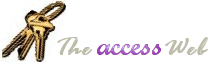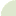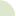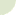Home  |   French  |   About  |   Search  | mvps.orgWhat's New Table Of Contents Credits Netiquette 10 Commandments  Bugs Tables Queries Forms Reports Modules APIs Strings Date/Time General Downloads Resources Search Feedback mvps.orgGeneral: Validate an IP Address Author(s) Paul OverwayHere is a snippet of code for validating an IP address. It is a string function that assures that the IP address is correctly formed. ```' ***** Code Start ***** Function IsValidIP(Arg As String) As Boolean On Error Resume Next Dim i As Integer Dim intCount As Integer Dim strTemp As String Dim strOut As String Dim strToken As String 'Too many or not enough characters If Len(Arg) > 15 Or Len(Arg) < 7 Then Arg = "" Exit Function End If For i = 1 To Len(Arg) If Mid(Arg, i, 1) = "." Then intCount = intCount + 1 End If 'Bail if bad character If InStr("1234567890.", Mid(Arg, i, 1)) = 0 Then Arg = "" Exit Function End If Next 'Not enough periods If intCount <> 3 Then Arg = "" Exit Function End If 'Can't start with period If Mid(Arg, 1, 1) = "." Then Arg = "" Exit Function End If strTemp = Arg For i = 1 To 4 If InStr(strTemp, ".") Then strToken = Mid(strTemp, 1, InStr(strTemp, ".") - 1) Else strToken = strTemp End If 'Empty token...bail If Len(strToken) = 0 Then Arg = "" Exit Function End If If CInt(strToken) > 255 Then 'Token value too high Arg = "" Exit Function Else 'OK strOut = strOut & CInt(strToken) & "." End If 'Lop it for next part If InStr(strTemp, ".") Then strTemp = Mid(strTemp, InStr(strTemp, ".") + 1) End If Next strOut = Left(strOut, Len(strOut) - 1) If strOut = Arg Then IsValidIP = True Else 'Arg was bad, but fixable. Return corrected version of Arg Arg = strOut IsValidIP = False End If End Function ' ***** Code End *****```© 1998-2010, Dev Ashish & Arvin Meyer, All rights reserved. Optimized for Microsoft Internet Explorer